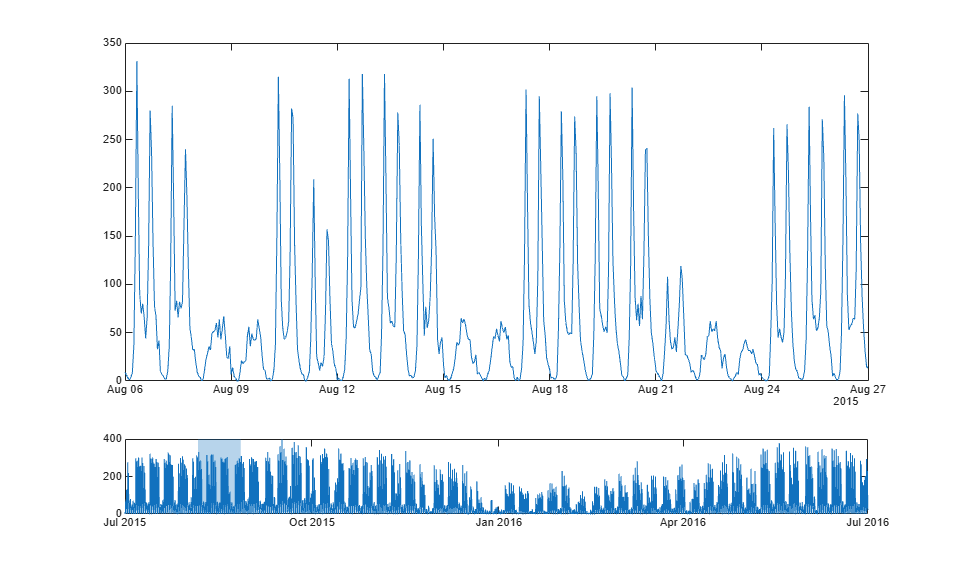# Chart Class Containing Two Interactive Plots

This example shows how to define a class for visualizing timetable data using two axes with interactive features. The top axes has panning and zooming enabled along the x dimension, so the user can examine a region of interest. The bottom axes displays a plot over the entire time range. The bottom axes also displays a light blue time window, which indicates the time range in the top axes. The class defines the following properties, methods, and local functions.

Properties:

• `Data` - A public and dependent property that stores a timetable.

• `TimeLimits` - A public property that sets the limits of the top axes and the width of the time window in the bottom axes.

• `SavedData` - A protected property that enables the user to save and load instances of the chart and preserve the data.

• `TopAxes` and `BottomAxes` - Private properties that store the axes objects.

• `TopLine` and `BottomLine` - Private properties that store the line objects.

• `TimeWindow` - A patch object displayed in the bottom axes, which indicates the time range of the top axes.

Methods:

• `set.Data` and `get.Data` - Enable the user to save and load instances of the chart and preserve the data.

• `setup` - Executes once when the chart is created. It configures the layout and the axes, the line objects, and the patch object.

• `update` - Executes after the `setup` method and after the user changes one or more properties on the chart.

• `panZoom` - Updates the time limits of the chart when the user pans or zooms within the top axes. This causes the time window to update to reflect the new limits.

• `click` - Recalculates the time limits when the user clicks the bottom axes.

Local Functions:

• `updateDataTipTemplate` - Called from within in the `update` method. It creates rows in the data tips that correspond to the variables in the timetable.

• `mustHaveOneNumericVariable` - Validates the `Data` property. This function ensures that the timetable specified by the user has at least one numeric variable.

To define the class, copy the following code into the editor and save it with the name `TimeTableChart.m` in a writable folder.

```classdef TimeTableChart < matlab.graphics.chartcontainer.ChartContainer properties (Dependent) Data timetable {mustHaveOneNumericVariable} = ... timetable(datetime.empty(0,1),zeros(0,1)) end properties TimeLimits (1,2) datetime = [NaT NaT] end properties (Access = protected) SavedData timetable = timetable(datetime.empty(0,1),zeros(0,1)) end properties (Access = private, Transient, NonCopyable) TopAxes matlab.graphics.axis.Axes TopLine matlab.graphics.chart.primitive.Line BottomAxes matlab.graphics.axis.Axes BottomLine matlab.graphics.chart.primitive.Line TimeWindow matlab.graphics.primitive.Patch end methods function set.Data(obj, tbl) % Reset the time limits if the row times have changed. oldTimes = obj.SavedData.Properties.RowTimes; newTimes = tbl.Properties.RowTimes; if ~isequal(oldTimes, newTimes) obj.TimeLimits = [NaT NaT]; end % Store the new table. obj.SavedData = tbl; end function tbl = get.Data(obj) tbl = obj.SavedData; end end methods (Access = protected) function setup(obj) % Create two axes. The top axes is 3x taller than bottom axes. tcl = getLayout(obj); tcl.GridSize = [4 1]; obj.TopAxes = nexttile(tcl, 1, [3 1]); obj.BottomAxes = nexttile(tcl, 4); % Add a shared toolbar on the layout, which removes the % toolbar from the individual axes. axtoolbar(tcl, 'default'); % Create one line to show the zoomed-in data. obj.TopLine = plot(obj.TopAxes, NaT, NaN); % Create one line to show an overview of the data, and disable % HitTest so the ButtonDownFcn on the bottom axes works. obj.BottomLine = plot(obj.BottomAxes, NaT, NaN, ... 'HitTest', 'off'); % Create a patch to show the current time limits. obj.TimeWindow = patch(obj.BottomAxes, ... 'Faces', 1:4, ... 'Vertices', NaN(4,2), ... 'FaceColor', obj.TopLine.Color, ... 'FaceAlpha', 0.3, ... 'EdgeColor', 'none', ... 'HitTest', 'off'); % Constrain axes panning/zooming to only the X-dimension. obj.TopAxes.Interactions = [ ... dataTipInteraction; panInteraction('Dimensions','x'); rulerPanInteraction('Dimensions','x'); zoomInteraction('Dimensions','x')]; % Disable pan/zoom on the bottom axes. obj.BottomAxes.Interactions = []; % Add a listener to XLim to respond to zoom events. addlistener(obj.TopAxes, 'XLim', 'PostSet', @(~, ~) panZoom(obj)); % Add a callback for clicks on the bottom axes. obj.BottomAxes.ButtonDownFcn = @(~, ~) click(obj); end function update(obj) % Extract the time data from the table. tbl = obj.Data; t = tbl.Properties.RowTimes; % Extract the numeric variables from the table. S = vartype('numeric'); numericTbl = tbl(:,S); % Update the data on both lines. set([obj.BottomLine obj.TopLine], 'XData', t, 'YData', numericTbl{:,1}); % Create a dataTipTextRow for each variable in the timetable. updateDataTipTemplate(obj.TopLine, tbl) % Update the top axes limits. obj.TopAxes.YLimMode = 'auto'; if obj.TimeLimits(1) < obj.TimeLimits(2) obj.TopAxes.XLim = obj.TimeLimits; else % Current time limits are invalid, so set XLimMode to auto and % let the axes calculate limits based on available data. obj.TopAxes.XLimMode = 'auto'; obj.TimeLimits = obj.TopAxes.XLim; end % Update time window to reflect the new time limits. xLimits = ruler2num(obj.TimeLimits, obj.BottomAxes.XAxis); yLimits = obj.BottomAxes.YLim; obj.TimeWindow.Vertices = [xLimits([1 1 2 2]); yLimits([1 2 2 1])]'; end function panZoom(obj) % When XLim on the top axes changes, update the time limits. obj.TimeLimits = obj.TopAxes.XLim; end function click(obj) % When clicking on the bottom axes, recenter the time limits. % Find the center of the click using CurrentPoint. center = obj.BottomAxes.CurrentPoint(1,1); % Convert from numeric units into datetime using num2ruler. center = num2ruler(center, obj.BottomAxes.XAxis); % Find the width of the current time limits. width = diff(obj.TimeLimits); % Recenter the current time limits. obj.TimeLimits = center + [-1 1]*width/2; end end end function updateDataTipTemplate(obj, tbl) % Create a dataTipTextRow for each variable in the timetable. timeVariable = tbl.Properties.DimensionNames{1}; rows = dataTipTextRow(timeVariable, tbl.(timeVariable)); for n = 1:numel(tbl.Properties.VariableNames) rows(n+1,1) = dataTipTextRow(... tbl.Properties.VariableNames{n}, tbl{:,n}); end obj.DataTipTemplate.DataTipRows = rows; end function mustHaveOneNumericVariable(tbl) % Validation function for Data property. S = vartype('numeric'); if width(tbl(:,S)) < 1 error('TimeTableChart:InvalidTable', ... 'Table must have at least one numeric variable.') end end ```

After saving the class file, create an instance of the chart. In this case, use the chart to examine a few weeks within a year of bicycle traffic data.

```bikeTbl = readtimetable('BicycleCounts.csv'); bikeTbl = bikeTbl(169:8954,:); tlimits = [datetime(2015,8,6) datetime(2015,8,27)]; TimeTableChart('Data',bikeTbl,'TimeLimits',tlimits); ```